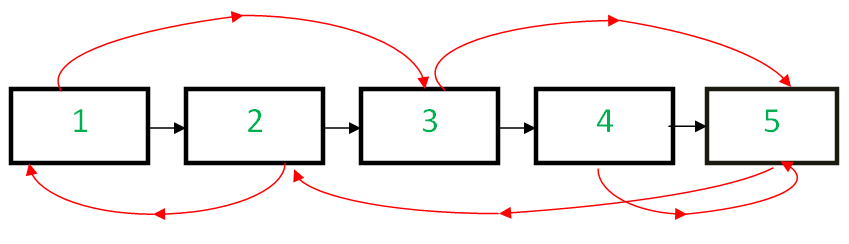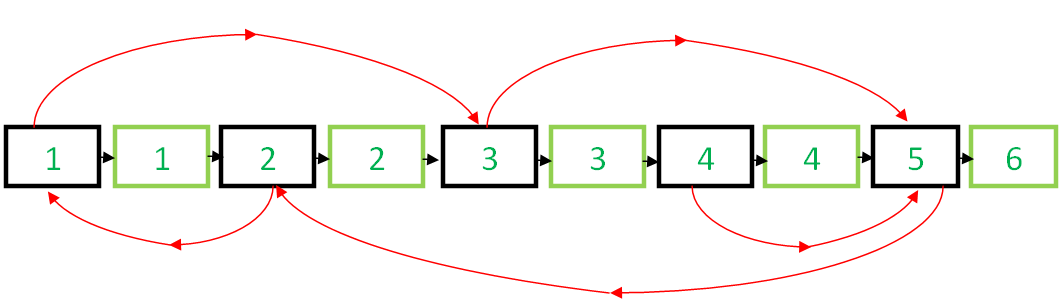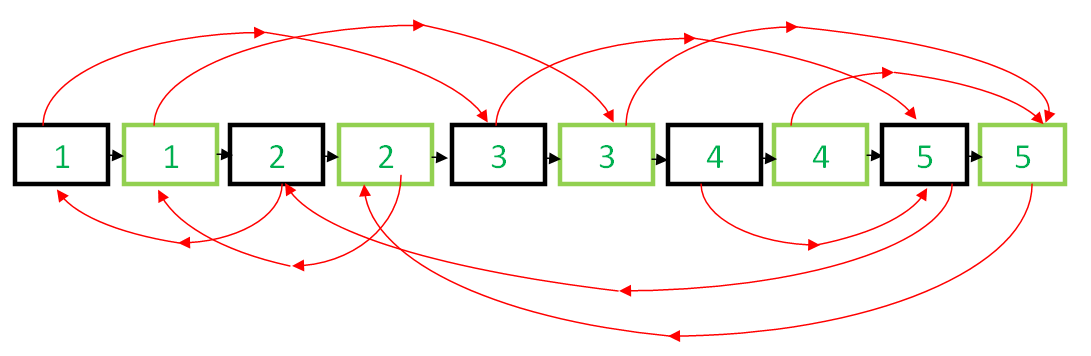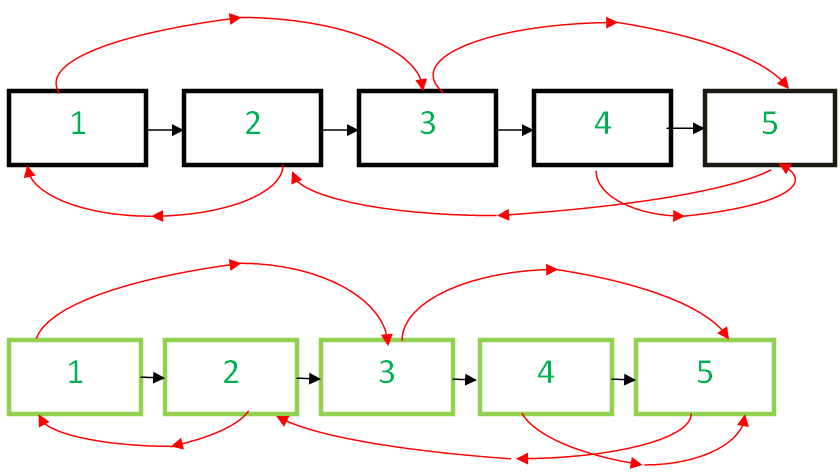Related Articles
Clone a linked list with next and random pointer in O(1) space
• Difficulty Level : Hard
• Last Updated : 03 Apr, 2020

Given a linked list having two pointers in each node. The first one points to the next node of the list, however, the other pointer is random and can point to any node of the list. Write a program that clones the given list in O(1) space, i.e., without any extra space.
Examples:

```Input : Head of the below-linked listOutput :
A new linked list identical to the original list.
```

## Recommended: Please try your approach on {IDE} first, before moving on to the solution.

In the previous posts Set-1 and Set-2 various methods are discussed, and O(n) space complexity implementation is also available.

In this post, we’ll be implementing an algorithm that’d require no additional space as discussed in Set-1.

Below is the Algorithm:

• Create the copy of node 1 and insert it between node 1 & node 2 in original Linked List, create a copy of 2 and insert it between 2 & 3. Continue in this fashion, add the copy of N after the Nth node• Now copy the random link in this fashion
``` original->next->random= original->random->next;  /*TRAVERSE
TWO NODES*/
```

This works because original->next is nothing but copy of original and Original->random->next is nothing but copy of random.• Now restore the original and copy linked lists in this fashion in a single loop.
```original->next = original->next->next;
copy->next = copy->next->next;```
• Ensure that original->next is NULL and return the cloned listBelow is the implementation.

## C++

 `// C++ program to clone a linked list with next``// and arbit pointers in O(n) time``#include ``using` `namespace` `std;`` ` `// Structure of linked list Node``struct` `Node``{``    ``int` `data;``    ``Node *next,*random;``    ``Node(``int` `x)``    ``{``        ``data = x;``        ``next = random = NULL;``    ``}``};`` ` `// Utility function to print the list.``void` `print(Node *start)``{``    ``Node *ptr = start;``    ``while` `(ptr)``    ``{``        ``cout << ``"Data = "` `<< ptr->data << ``", Random  = "``             ``<< ptr->random->data << endl;``        ``ptr = ptr->next;``    ``}``}`` ` `// This function clones a given linked list``// in O(1) space``Node* clone(Node *start)``{``    ``Node* curr = start, *temp;`` ` `    ``// insert additional node after``    ``// every node of original list``    ``while` `(curr)``    ``{``        ``temp = curr->next;`` ` `        ``// Inserting node``        ``curr->next = ``new` `Node(curr->data);``        ``curr->next->next = temp;``        ``curr = temp;``    ``}`` ` `    ``curr = start;`` ` `    ``// adjust the random pointers of the``    ``// newly added nodes``    ``while` `(curr)``    ``{``        ``if``(curr->next)``            ``curr->next->random = curr->random ? ``                                 ``curr->random->next : curr->random;`` ` `        ``// move to the next newly added node by``        ``// skipping an original node``        ``curr = curr->next?curr->next->next:curr->next;``    ``}`` ` `    ``Node* original = start, *copy = start->next;`` ` `    ``// save the start of copied linked list``    ``temp = copy;`` ` `    ``// now separate the original list and copied list``    ``while` `(original && copy)``    ``{``        ``original->next =``         ``original->next? original->next->next : original->next;`` ` `        ``copy->next = copy->next?copy->next->next:copy->next;``        ``original = original->next;``        ``copy = copy->next;``    ``}`` ` `    ``return` `temp;``}`` ` `// Driver code``int` `main()``{``    ``Node* start = ``new` `Node(1);``    ``start->next = ``new` `Node(2);``    ``start->next->next = ``new` `Node(3);``    ``start->next->next->next = ``new` `Node(4);``    ``start->next->next->next->next = ``new` `Node(5);`` ` `    ``// 1's random points to 3``    ``start->random = start->next->next;`` ` `    ``// 2's random points to 1``    ``start->next->random = start;`` ` `    ``// 3's and 4's random points to 5``    ``start->next->next->random =``                    ``start->next->next->next->next;``    ``start->next->next->next->random =``                    ``start->next->next->next->next;`` ` `    ``// 5's random points to 2``    ``start->next->next->next->next->random =``                                      ``start->next;`` ` `    ``cout << ``"Original list : \n"``;``    ``print(start);`` ` `    ``cout << ``"\nCloned list : \n"``;``    ``Node *cloned_list = clone(start);``    ``print(cloned_list);`` ` `    ``return` `0;``}`

## Java

 `// Java program to clone a linked list with next ``// and arbit pointers in O(n) time ``class` `GfG``{ `` ` `// Structure of linked list Node ``static` `class` `Node ``{ ``    ``int` `data; ``    ``Node next,random; ``    ``Node(``int` `x) ``    ``{ ``        ``data = x; ``        ``next = random = ``null``; ``    ``} ``}`` ` `// Utility function to print the list. ``static` `void` `print(Node start) ``{ ``    ``Node ptr = start; ``    ``while` `(ptr != ``null``) ``    ``{ ``        ``System.out.println(``"Data = "` `+ ptr.data +``                    ``", Random = "``+ptr.random.data); ``        ``ptr = ptr.next; ``    ``} ``} `` ` `// This function clones a given  ``// linked list in O(1) space ``static` `Node clone(Node start) ``{ ``    ``Node curr = start, temp = ``null``; `` ` `    ``// insert additional node after ``    ``// every node of original list ``    ``while` `(curr != ``null``) ``    ``{ ``        ``temp = curr.next; `` ` `        ``// Inserting node ``        ``curr.next = ``new` `Node(curr.data); ``        ``curr.next.next = temp; ``        ``curr = temp; ``    ``} ``    ``curr = start; `` ` `    ``// adjust the random pointers of the ``    ``// newly added nodes ``    ``while` `(curr != ``null``) ``    ``{ ``        ``if``(curr.next != ``null``) ``            ``curr.next.random = (curr.random != ``null``)``                      ``? curr.random.next : curr.random; `` ` `        ``// move to the next newly added node by ``        ``// skipping an original node ``        ``curr = (curr.next != ``null``) ? curr.next.next ``                                        ``: curr.next; ``    ``} `` ` `    ``Node original = start, copy = start.next; `` ` `    ``// save the start of copied linked list ``    ``temp = copy; `` ` `    ``// now separate the original list and copied list ``    ``while` `(original != ``null` `&& copy != ``null``) ``    ``{ ``        ``original.next = (original.next != ``null``)? ``                    ``original.next.next : original.next; `` ` `        ``copy.next = (copy.next != ``null``) ? copy.next.next ``                                            ``: copy.next; ``        ``original = original.next; ``        ``copy = copy.next; ``    ``} ``    ``return` `temp; ``} `` ` `// Driver code ``public` `static` `void` `main(String[] args) ``{ ``    ``Node start = ``new` `Node(``1``); ``    ``start.next = ``new` `Node(``2``); ``    ``start.next.next = ``new` `Node(``3``); ``    ``start.next.next.next = ``new` `Node(``4``); ``    ``start.next.next.next.next = ``new` `Node(``5``); `` ` `    ``// 1's random points to 3 ``    ``start.random = start.next.next; `` ` `    ``// 2's random points to 1 ``    ``start.next.random = start; `` ` `    ``// 3's and 4's random points to 5 ``    ``start.next.next.random = start.next.next.next.next; ``    ``start.next.next.next.random = start.next.next.next.next; `` ` `    ``// 5's random points to 2 ``    ``start.next.next.next.next.random = start.next; `` ` `    ``System.out.println(``"Original list : "``); ``    ``print(start); `` ` `    ``System.out.println(``"Cloned list : "``); ``    ``Node cloned_list = clone(start); ``    ``print(cloned_list); `` ` `}``} `` ` `// This code is contributed by Prerna Saini.`

## C#

 `// C# program to clone a linked list with ``// next and arbit pointers in O(n) time ``using` `System;`` ` `class` `GFG ``{ `` ` `// Structure of linked list Node ``class` `Node ``{ ``    ``public` `int` `data; ``    ``public` `Node next, random; ``    ``public` `Node(``int` `x) ``    ``{ ``        ``data = x; ``        ``next = random = ``null``; ``    ``} ``} `` ` `// Utility function to print the list. ``static` `void` `print(Node start) ``{ ``    ``Node ptr = start; ``    ``while` `(ptr != ``null``) ``    ``{ ``        ``Console.WriteLine(``"Data = "` `+ ptr.data + ``                          ``", Random = "` `+``                          ``ptr.random.data); ``        ``ptr = ptr.next; ``    ``} ``} `` ` `// This function clones a given ``// linked list in O(1) space ``static` `Node clone(Node start) ``{ ``    ``Node curr = start, temp = ``null``; `` ` `    ``// insert additional node after ``    ``// every node of original list ``    ``while` `(curr != ``null``) ``    ``{ ``        ``temp = curr.next; `` ` `        ``// Inserting node ``        ``curr.next = ``new` `Node(curr.data); ``        ``curr.next.next = temp; ``        ``curr = temp; ``    ``} ``    ``curr = start; `` ` `    ``// adjust the random pointers of ``    ``// the newly added nodes ``    ``while` `(curr != ``null``) ``    ``{ ``        ``if``(curr.next != ``null``) ``            ``curr.next.random = (curr.random != ``null``) ``                    ``? curr.random.next : curr.random; `` ` `        ``// move to the next newly added node ``        ``// by skipping an original node ``        ``curr = (curr.next != ``null``) ? curr.next.next ``                                        ``: curr.next; ``    ``} `` ` `    ``Node original = start, copy = start.next; `` ` `    ``// save the start of copied linked list ``    ``temp = copy; `` ` `    ``// now separate the original list ``    ``// and copied list ``    ``while` `(original != ``null` `&& copy != ``null``) ``    ``{ ``        ``original.next = (original.next != ``null``)? ``                    ``original.next.next : original.next; `` ` `        ``copy.next = (copy.next != ``null``) ? copy.next.next ``                                             ``: copy.next; ``        ``original = original.next; ``        ``copy = copy.next; ``    ``} ``    ``return` `temp; ``} `` ` `// Driver code ``public` `static` `void` `Main(String[] args) ``{ ``    ``Node start = ``new` `Node(1); ``    ``start.next = ``new` `Node(2); ``    ``start.next.next = ``new` `Node(3); ``    ``start.next.next.next = ``new` `Node(4); ``    ``start.next.next.next.next = ``new` `Node(5); `` ` `    ``// 1's random points to 3 ``    ``start.random = start.next.next; `` ` `    ``// 2's random points to 1 ``    ``start.next.random = start; `` ` `    ``// 3's and 4's random points to 5 ``    ``start.next.next.random = start.next.next.next.next; ``    ``start.next.next.next.random = start.next.next.next.next; `` ` `    ``// 5's random points to 2 ``    ``start.next.next.next.next.random = start.next; `` ` `    ``Console.WriteLine(``"Original list : "``); ``    ``print(start); `` ` `    ``Console.WriteLine(``"Cloned list : "``); ``    ``Node cloned_list = clone(start); ``    ``print(cloned_list); ``} ``} `` ` `// This code has been contributed ``// by Rajput-Ji`

## Python

 `'''Python program to clone a linked list with next and arbitrary pointers'''``'''Done in O(n) time with O(1) extra space'''`` ` `class` `Node:``    ``'''Structure of linked list node'''`` ` `    ``def` `__init__(``self``, data):``        ``self``.data ``=` `data``        ``self``.``next` `=` `None``        ``self``.random ``=` `None`` ` `def` `clone(original_root):``    ``'''Clone a doubly linked list with random pointer'''``    ``'''with O(1) extra space'''`` ` `    ``'''Insert additional node after every node of original list'''``    ``curr ``=` `original_root``    ``while` `curr !``=` `None``:``        ``new ``=` `Node(curr.data)``        ``new.``next` `=` `curr.``next``        ``curr.``next` `=` `new``        ``curr ``=` `curr.``next``.``next`` ` `    ``'''Adjust the random pointers of the newly added nodes'''``    ``curr ``=` `original_root``    ``while` `curr !``=` `None``:``        ``curr.``next``.random ``=` `curr.random.``next``        ``curr ``=` `curr.``next``.``next`` ` `    ``'''Detach original and duplicate list from each other'''``    ``curr ``=` `original_root``    ``dup_root ``=` `original_root.``next``    ``while` `curr.``next` `!``=` `None``:``        ``tmp ``=` `curr.``next``        ``curr.``next` `=` `curr.``next``.``next``        ``curr ``=` `tmp`` ` `    ``return` `dup_root`` ` `def` `print_dlist(root):``    ``'''Function to print the doubly linked list'''`` ` `    ``curr ``=` `root``    ``while` `curr !``=` `None``:``        ``print``(``'Data ='``, curr.data, ``', Random ='``, curr.random.data)``        ``curr ``=` `curr.``next`` ` `####### Driver code #######``'''Create a doubly linked list'''``original_list ``=` `Node(``1``)``original_list.``next` `=` `Node(``2``)``original_list.``next``.``next` `=` `Node(``3``)``original_list.``next``.``next``.``next` `=` `Node(``4``)``original_list.``next``.``next``.``next``.``next` `=` `Node(``5``)`` ` `'''Set the random pointers'''`` ` `# 1's random points to 3``original_list.random ``=` `original_list.``next``.``next`` ` `# 2's random points to 1``original_list.``next``.random ``=` `original_list`` ` `# 3's random points to 5``original_list.``next``.``next``.random ``=` `original_list.``next``.``next``.``next``.``next`` ` `# 4's random points to 5``original_list.``next``.``next``.``next``.random ``=` `original_list.``next``.``next``.``next``.``next`` ` `# 5's random points to 2``original_list.``next``.``next``.``next``.``next``.random ``=` `original_list.``next`` ` `'''Print the original linked list'''``print``(``'Original list:'``)``print_dlist(original_list)`` ` `'''Create a duplicate linked list'''``cloned_list ``=` `clone(original_list)`` ` `'''Print the duplicate linked list'''``print``(``'\nCloned list:'``)``print_dlist(cloned_list)`` ` `'''This code is contributed by Shashank Singh'''`

Output:
```Original list :
Data = 1, Random  = 3
Data = 2, Random  = 1
Data = 3, Random  = 5
Data = 4, Random  = 5
Data = 5, Random  = 2

Cloned list :
Data = 1, Random  = 3
Data = 2, Random  = 1
Data = 3, Random  = 5
Data = 4, Random  = 5
Data = 5, Random  = 2
```

This article is contributed by Ashutosh Kumar 😀 If you like GeeksforGeeks and would like to contribute, you can also write an article using contribute.geeksforgeeks.org or mail your article to contribute@geeksforgeeks.org. See your article appearing on the GeeksforGeeks main page and help other Geeks.

Please write comments if you find anything incorrect, or you want to share more information about the topic discussed above.

Attention reader! Don’t stop learning now. Get hold of all the important DSA concepts with the DSA Self Paced Course at a student-friendly price and become industry ready.

My Personal Notes arrow_drop_up